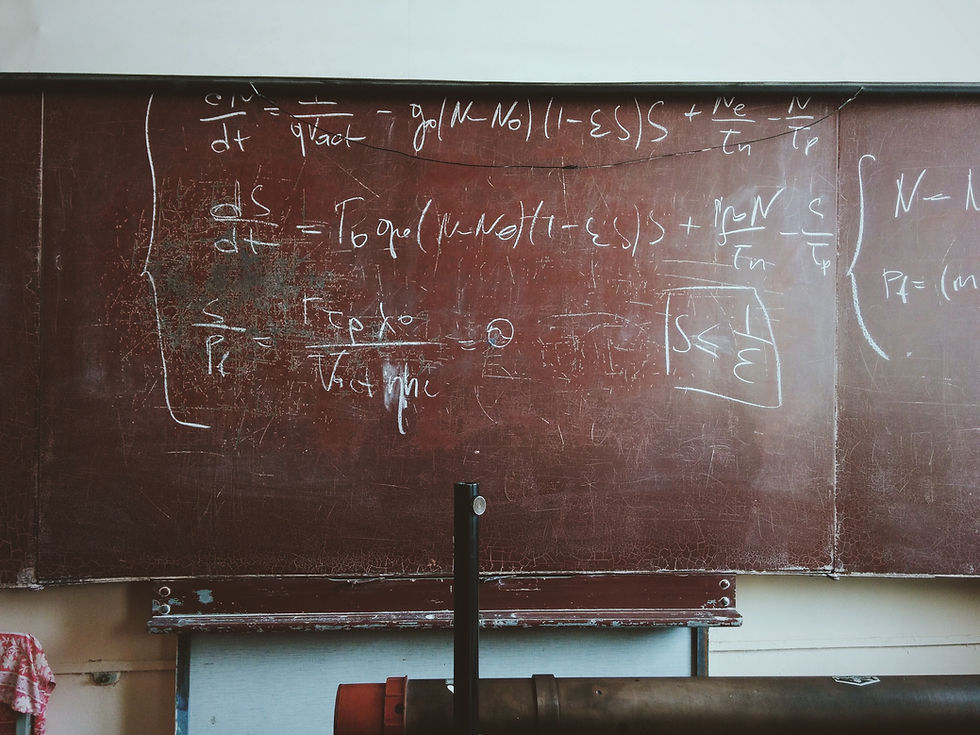top of page
Search
• Benjamin Lam

# Students: What is differentiation and why is learning differentiation important?

Differentiation is a powerful tool used in mathematics to find the rate of change between two variables. It is an important concept that can be used to solve many problems, from finding the slope of a line to calculating the area under a curve. Differentiation can also be used to optimize functions and find maximum or minimum values. In this article, we will explore what differentiation is and how it can be applied in various contexts.

1. Differentiation can be used to find the instantaneous rate of change of a function at any given point. This is useful for understanding how a function behaves in certain areas and can help identify local extrema (maximums and minimums).

2. Differentiation can also be used to calculate the area under a curve, which is important for calculating integrals.

3. Differentiation can be used to optimize functions by finding the maximum or minimum values of a function. This is useful for finding the most efficient solution to a problem or maximizing profits in business applications.

4. Differentiation can also be used to solve differential equations, which are equations that involve derivatives of functions with respect to one or more variables.

5. Differentiation can be used to find the tangent line of a curve at any given point, which is useful for understanding how a function behaves in certain areas.

6. Differentiation can also be used to calculate the curvature of a curve, which is important for understanding the shape of a function and its behaviour in certain areas.

7. Finally, differentiation can be used to solve optimization problems, such as finding the shortest path between two points or the most efficient way to allocate resources.

## Why is learning differentiation in school important?Learning differentiation in school is important because it provides a foundation for understanding more advanced mathematical concepts. Differentiation can be used to solve many real-world problems, such as finding the maximum profit in a business or calculating the area under a curve. It is also an essential tool for calculus and other higher level mathematics courses. By learning differentiation, students gain an understanding of how functions behave and how to optimize them . This knowledge can be applied to many different areas, from engineering to economics.

## What are the different methods used in differentiation?

The most common methods used in differentiation are the power rule, the product rule, the quotient rule, and the chain rule. The power rule states that if a function is raised to a certain power, then its derivative is equal to that power multiplied by the original function raised to one less than that power. The product rule states that if two functions are multiplied together, then their derivatives can be found

## Why is differentiation so difficult?

Differentiation can be difficult because it involves understanding how a function behaves in different areas and how the variables interact with each other. It also requires being able to identify local extrema (maximums and minimums) and calculate derivatives accurately. Additionally, some problems may require multiple steps or involve complex equations, which can make them more challenging to solve.

## Is it normal for students to struggle with differentiation?

Yes, it is normal for students to struggle with differentiation. Differentiation can be a difficult concept to understand and requires a lot of practice to master. Additionally, some problems may require multiple steps or involve complex equations, which can make them even more challenging. However, with enough practice and dedication, most students should be able to eventually understand and master the concepts of differentiation.

## How can I make differentiation easier?

One way to make differentiation easier is to practice solving problems. Working through examples and doing practice problems can help you understand the concepts and develop your skills. Additionally, it can be helpful to break down difficult problems into smaller parts, as this can make them easier to solve. Finally, using visual aids such as graphs or diagrams can help you visualize the problem and better understand how the variables interact with each other.

## What are some real life uses of differentiation?

Differentiation has many real-life applications. In economics, it can be used to calculate the marginal cost of production or the marginal revenue of a product. In engineering, it can be used to optimize designs and find the most efficient way to allocate resources. Differentiation is also used in physics to calculate velocity, acceleration, and other properties of motion. Finally, differentiation can be used in biology to study the growth of populations and the spread of diseases.

## Conclusion

In conclusion, differentiation is an important mathematical concept that has many real-world applications. It can be used to solve problems in economics, engineering, physics, and biology. Differentiation can be difficult to understand at first, but with practice and the use of visual aids, it can become easier. Understanding differentiation is essential for higher level mathematics courses and provides a foundation for more advanced concepts.